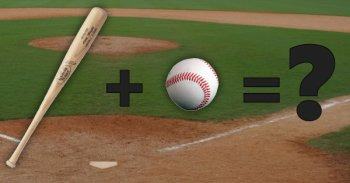Games
Problems
Go Pro!

A video has been floating around that talks about "fast thinking" and "slow thinking," and a simple three-question test that people who perform well on tend to do better on a lot of other tasks. Here are the three questions:1. A baseball bat costs a dollar more than a baseball. Together they cost \$1.10. What is the cost of the baseball?

2.  If it takes 5 machines 5 minutes to make 5 widgets, how long would it take 100 machines to make 100 widgets?

3.  In a lake, there is a patch of lily pads.Every day the patch doubles in size. If it takes 48 days for the patch to completely cover the lake, how long would it take to cover half the lake?

The video doesn't give answers or explain all the questions. Can you help?

I'll give it a go. But first, let me guess what the "fast thinking" (incorrect) answers are:

1.  The ball costs ten cents.

2.  It takes 100 minutes.

3.  It takes 24 days.

Were those the answers you came up with? If they were, don't feel bad - these are the "natural" answers. But let's look at the real answers.

## Baseball Problem

If b is the cost of the baseball, then the cost of the baseball bat is b + 1.  This means that b + (b + 1) = \$1.10. This leads us to 2b + 1 = 1.1, or 2b = .1. Dividing both sides by 2 gives us b = \$0.05, or five cents. This is half of the instinctive response.

## Widget Problem

You have 20 times as many machines, which means they can make 20 times as many widgets in five minutes. You know what that means, right? It takes 5 minutes!  All 100 of those machines are working simultaneously, and each group of 5 machines makes 5 widgets in that five minutes.  This is similar to the cat and mouse problem which can be found in our reference pages: Cat and Mouse Variation.Like us on Facebook to get updates about new resources